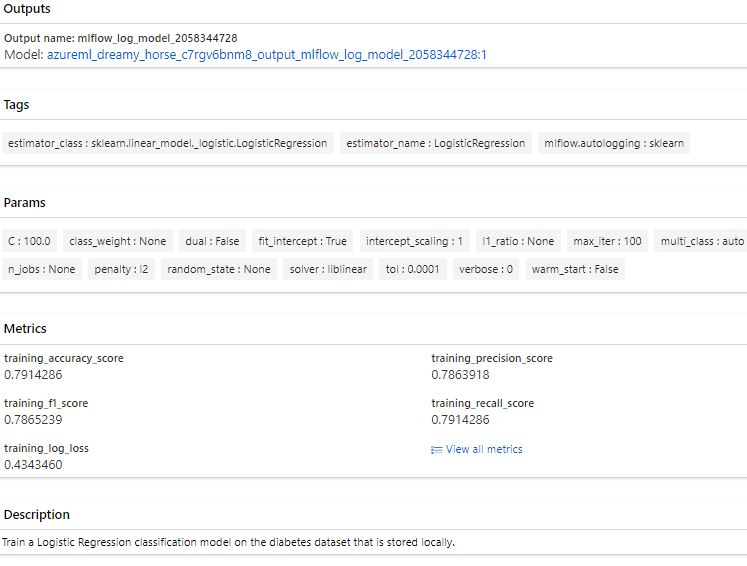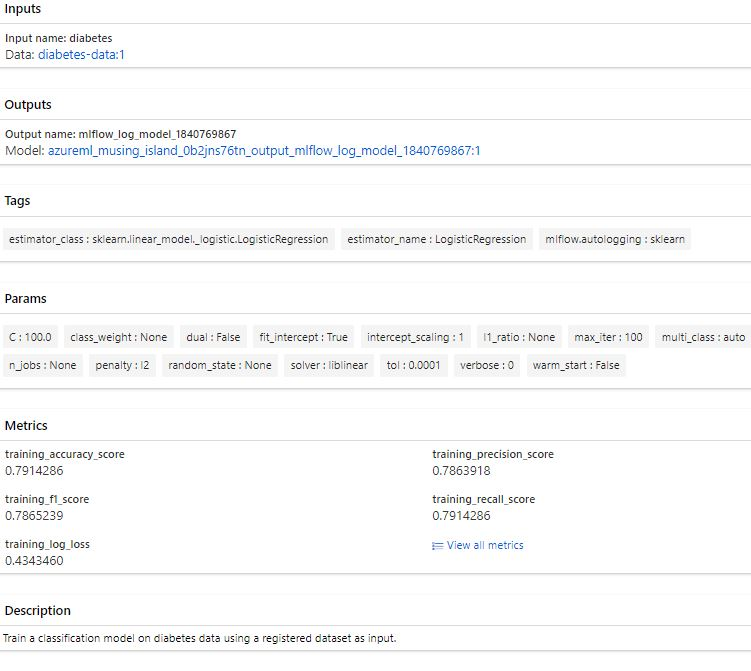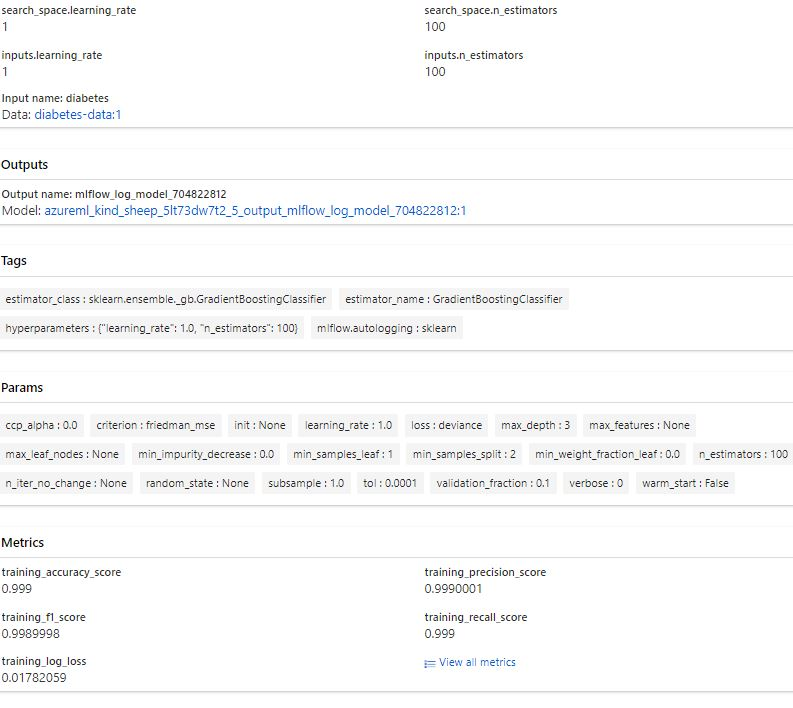# Visual Studio code - Azure CLI - ML manage jobs

Rating & reviews (0 reviews)
Study notes

Setup VS code for ML Jobs: Visual Studio code - Azure CLI basic (cristinabrata.com)
Summary
VS code has set the default Azure Workspace and Environment
1. Script
2. Environment
3. Compute
Create environment.
You expect to use a compute cluster in the future to retrain the model whenever needed. To train the model on either a compute instance or compute cluster, all necessary packages need to be installed on the compute to run the code. Instead of manually installing these packages every time you use a new compute, you can list them in an environment.
Every Azure Machine Learning workspace will by default have a list of curated environments when you create the workspace. Curated environments include common machine learning packages to train a model.

Necessary two files a .py and a .yml (in the same folder for this example):

basic-env-ml.yml
name: basic-env-ml
channels:
- conda-forge
dependencies:
- python=3.8
- pip
- pip:
- numpy
- pandas
- scikit-learn
- matplotlib
- azureml-mlflow
basic-env.yml

\$schema: https://azuremlschemas.azureedge.net/latest/environment.schema.json
name: basic-env-scikit
version: 1
image: mcr.microsoft.com/azureml/openmpi3.1.2-ubuntu18.04
conda_file: file:conda-envs/basic-env-ml.yml
Run
az ml environment create --file ./PATH_TO_YAML_FILE/basic-env.yml

Train the model (Create Azure ML job)
Theer are one .yaml and one .py files

main.py
The code that generate the experiment / job.
All in here will be exceuted in Azure on the instance/cluster set in data_job.yaml
Immediately job is created, it starts.

# Import libraries
import mlflow
import argparse

import pandas as pd
from sklearn.model_selection import train_test_split
from sklearn.linear_model import LogisticRegression

# define functions
def main(args):
# enable auto logging
mlflow.autolog()
# process data
X_train, X_test, y_train, y_test = process_data(df)

# train model
model = train_model(args.reg_rate, X_train, X_test, y_train, y_test)
def process_data(df):
# split dataframe into X and y
X, y = df[['Pregnancies','PlasmaGlucose','DiastolicBloodPressure','TricepsThickness','SerumInsulin','BMI','DiabetesPedigree','Age']].values, df['Diabetic'].values

# train/test split
X_train, X_test, y_train, y_test = train_test_split(X, y, test_size=0.30, random_state=0)

# return splits and encoder
return X_train, X_test, y_train, y_test

def train_model(reg_rate, X_train, X_test, y_train, y_test):
# train model
model = LogisticRegression(C=1/reg_rate, solver="liblinear").fit(X_train, y_train)

# return model
return model

def parse_args():
# setup arg parser
parser = argparse.ArgumentParser()

# parse args
args = parser.parse_args()

# return args
return args

# run script
if __name__ == "__main__":
print(" ")
print("*" * 60)

# parse args
args = parse_args()

# run main function
main(args)

print("*" * 60)
print(" ")

data_job.yml
This is the file run in CLI
\$schema: https://azuremlschemas.azureedge.net/latest/commandJob.schema.json
# folder where is main_py
code: src
command: >-
python main.py
--diabetes-csv \${{inputs.diabetes}}
inputs:
diabetes:
path: azureml:diabetes-data:1
mode: ro_mount
environment: azureml:basic-env-scikit@latest
# It is created and running (if stoped - job will be set in queue
compute: azureml:COMPUTE_INSTANCE_OR_CLUSTER
experiment_name: diabetes-data-example
description: Train a classification model on diabetes data using a registered dataset as input.

Run
# When you include the parameter --web, a web page will open after the job is submitted so you can monitor the experiment run in the Azure Machine Learning Studio.
az ml job create --file ./PATH_TO_YAML_FILE/basic-job.yml --web

We can se job in Azure ML Studio.Add dataset as input to job

Important is to retrain the model and from time to time use a new dataset to keep it up to date and make it better.
To easily change the input dataset every time you want to retrain the model, you want to create an input argument for the data.
Replace locally stored CSV (from the training script) with an YAML file.
1. In the script
You define the input arguments using the argparse module. You specify the argument's name, type and optionally a default value.
2. In the YAML file:
You specify the data input, which will mount (default option) or download data to the local file system. You can refer to a public URI or a registered dataset in the Azure Machine Learning workspace.

Train a new model:
There are one .yml and one .py files

main.py
The code that generate the experiment / job.
All in here will be exceuted in Azure on the instance/cluster set in data_job.yaml
Immediately job is created, it starts.

# Import libraries
import mlflow
import argparse
import glob

import pandas as pd
from sklearn.model_selection import train_test_split
from sklearn.linear_model import LogisticRegression

# IT WAS

# define functions
def main(args):
# enable auto logging
mlflow.autolog()

data_path = args.diabetes_csv
all_files = glob.glob(data_path + "/*.csv")
df = pd.concat((pd.read_csv(f) for f in all_files), sort=False)

# process data
X_train, X_test, y_train, y_test = process_data(df)

# train model
model = train_model(args.reg_rate, X_train, X_test, y_train, y_test)

def process_data(df):
# split dataframe into X and y
X, y = df[['Pregnancies','PlasmaGlucose','DiastolicBloodPressure','TricepsThickness','SerumInsulin','BMI','DiabetesPedigree','Age']].values, df['Diabetic'].values

# train/test split
X_train, X_test, y_train, y_test = train_test_split(X, y, test_size=0.30, random_state=0)

# return splits and encoder
return X_train, X_test, y_train, y_test

def train_model(reg_rate, X_train, X_test, y_train, y_test):
# train model
model = LogisticRegression(C=1/reg_rate, solver="liblinear").fit(X_train, y_train)

# return model
return model

def parse_args():
# setup arg parser
parser = argparse.ArgumentParser()

# parse args
args = parser.parse_args()

# return args
return args

# run script
if __name__ == "__main__":
print(" ")
print("*" * 60)

# parse args
args = parse_args()

# run main function
main(args)

print("*" * 60)
print(" ")

data_job.yml
This is the file run in CLI

\$schema: https://azuremlschemas.azureedge.net/latest/commandJob.schema.json
# folder where is main_py
code: src
command: >-
python main.py
# bellow is new
--diabetes-csv \${{inputs.diabetes}}
inputs:
diabetes:
path: azureml:diabetes-data:1
mode: ro_mount
# above is new
environment: azureml:basic-env-scikit@latest
# It is created and running (if stoped - job will be set in queue
compute: azureml:COMPUTE_INSTANCE_OR_CLUSTER
experiment_name: diabetes-data-example
description: Train a classification model on diabetes data using a registered dataset as input.

Run
# When you include the parameter --web, a web page will open after the job is submitted so you can monitor the experiment run in the Azure Machine Learning Studio.
az ml job create --file ./PATH_TO_YAML_FILE/data-job.yml

Result from Azure ML StudioRun a Job using hyperparameter (tune job)

Perform hyperparameter tuning with the Azure Machine Learning workspace by submitting a sweep job.
Use a sweep job to configure and submit a hyperparameter tuning job via the CLI (v2).

Hyperparametertuning allows to train multiple models, using the same algorithm and training data but different hyperparameter values.
For each iteration, the performance metrics need to be tracked to evaluate which configuration resulted in the best model.

Target - Instance cluster.

If you have note run:
az ml compute create --name "CLUSTER_NAME" --size STANDARD_DS11_V2 --max-instance 2 --type AmlCompute

There are two files; a .py and a .yml

main.py
Contains the python script that train the model (write and test in Jupyter note and pack it here)

import mlflow
import argparse
import glob

import pandas as pd
from sklearn.model_selection import train_test_split

# define functions
def main(args):
# enable auto logging
mlflow.autolog()
params = {
"learning_rate": args.learning_rate,
"n_estimators": args.n_estimators,
}

data_path = args.diabetes_csv
all_files = glob.glob(data_path + "/*.csv")
df = pd.concat((pd.read_csv(f) for f in all_files), sort=False)
# process data
X_train, X_test, y_train, y_test = process_data(df)

# train model
model = train_model(params, X_train, X_test, y_train, y_test)
def process_data(df):
# split dataframe into X and y
X, y = df[['Pregnancies','PlasmaGlucose','DiastolicBloodPressure','TricepsThickness','SerumInsulin','BMI','DiabetesPedigree','Age']].values, df['Diabetic'].values

# train/test split
X_train, X_test, y_train, y_test = train_test_split(X, y, test_size=0.30, random_state=0)

# return splits and encoder
return X_train, X_test, y_train, y_test

def train_model(params, X_train, X_test, y_train, y_test):
# train model
model = model.fit(X_train, y_train)

# return model
return model

def parse_args():
# setup arg parser
parser = argparse.ArgumentParser()

# parse args
args = parser.parse_args()

# return args
return args

# run script
if __name__ == "__main__":
print(" ")
print("*" * 60)

# parse args
args = parse_args()

# run main function
main(args)

print("*" * 60)
print(" ")

There are two hyperparameter values:
• Learning rate:
with search space [0.01, 0.1, 1.0]
• N estimators:
with search space [10, 100]
sweep-job.yml
File to run - creates experiemnt.

\$schema: https://azuremlschemas.azureedge.net/latest/sweepJob.schema.json
type: sweep
sampling_algorithm: grid
trial:
code: src
command: >-
python main.py
--diabetes-csv \${{inputs.diabetes}}
--learning-rate \${{search_space.learning_rate}}
--n-estimators \${{search_space.n_estimators}}
environment: azureml:basic-env-scikit@latest
inputs:
diabetes:
path: azureml:diabetes-data:1
mode: ro_mount
compute: azureml:CLUSTER_NAME
search_space:
learning_rate:
type: choice
values: [0.01, 0.1, 1.0]
n_estimators:
type: choice
values: [10, 100]
objective:
primary_metric: training_roc_auc_score
goal: maximize
limits:
max_total_trials: 6
max_concurrent_trials: 3
timeout: 3600
experiment_name: diabetes-sweep-example
description: Run a hyperparameter sweep job for classification on diabetes dataset.

Parameters:
• type:
The job type, which in this case is sweep_job.
• algorithm:
The sampling method used to choose values from the search space. Can be bayesian, grid, or random.
• search_space:
The set of values tried during hyperparameter tuning. For each hyperparameter, you can configure the search space type (choice) and values (0.01, 0.1, 1.0).
• objective:
The name of the logged metric that is used to decide which model is best (primary_metric). And whether that metric is best when maximized or minimized (goal).
• max_total_trials:
A hard stop for how many models to train in total.
• max_concurrent_trials:
When you use a compute cluster, you can train models in parallel. The number of maximum concurrent trials can't be higher than the number of nodes provisioned for the compute cluster.
Run to submit job:
az ml job create --file ./PATH_TO_JOB_FILE/sweep-job.yml

In Azure ML Studio
Best trial results:References:
Train models in Azure Machine Learning with the CLI (v2) - Training | Microsoft Learn
Visual Studio code - Azure CLI basic (cristinabrata.com)
Train ML models - Azure Machine Learning | Microsoft Learn
az ml job | Microsoft Learn
azureml-examples/cli at main · Azure/azureml-examples (github.com)